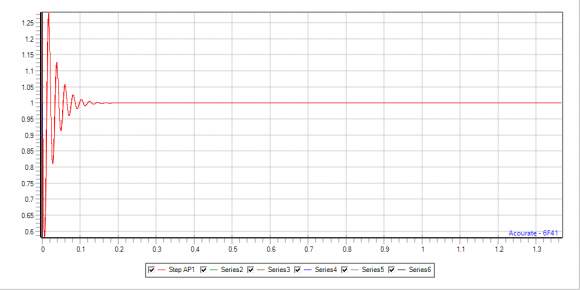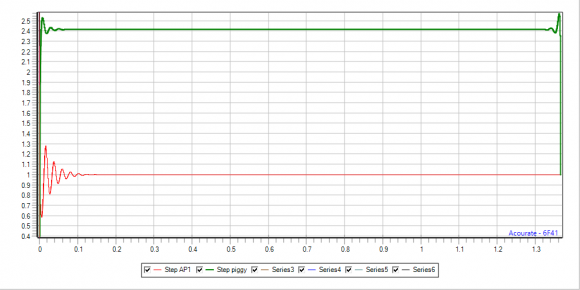## FIR allpass with desired group delayStarted by 4 years ago10 replieslatest reply 4 years ago255 views
Hello, I have a question about designing a reasonable FIR allpass filter with a desired group delay. Example: a FIR filter is designed as finite sequence from a 2nd order IIR allpass with f= 46.875 Hz, Q= 4 @ samplerate 48 kHz The max. group delay of the filter is about 54 ms, the curve is a bell-shaped curve. Now I like to create a reasonable allpass filter (causal, similar to minimum-phase) with a group delay of e.g. max. 10 ms. This can be done by reducing Q but this leads to a wider frequeny range compared to Q=4. So the new group delay should behave like the Q=4 delay but with delay e.g. target_gd = Q4_gd / 5.4 Is that possible? If yes, how? If not, why? Uli
[ - ]I think you're over-complicating this in your head. You can do this with a large fixed delay line. Just apply a delay of fs * T samples, where T is your delay in seconds, and that will perfectly achieve the group delay you want.

For example, if you want a group delay of 10 ms, just delay the signal by 480 samples and you're there.

[ - ]I'm sorry but you have not understood my question.

It is not dealing with a simple delay line with x samples. Such a delay line delays all frequencies equally.

But I like to get only a frequency certain range delayed. So e.g. think about delaying 100 Hz to 400 Hz by 10 ms. Whereas frequencies up to 100 Hz and above 400 Hz should have 0 delay.

Furthermore the amplitude should be left untouched = allpass behaviour.

Of course is is clear, that a sudden rise/stop of delay will cause trouble.

That's what I'm talking about a bell shaped curve as an example for smooth transition between 0 delay and desired delay.

A typical 2nd order allpass shows such a behaviour (and also a nice causal pulse response) but the delay is not really flexible. I like to control both width of frequency range and delay time.

So far I have not found a solution by e.g. design of magnitude/phase response, polar to cart conversion and IFFT.

[ - ]Okay, so if I understand you correctly, you are looking for an all-pass filter in magnitude, with a fixed group delay over some range of frequencies?

Because a constant group delay corresponds to a linear phase delay, that means that the phase response of your filter should be piecewise linear. That is, the phase should be constant up to your "delayband," have a linear phase with slope determined by your delay, then be constant again after.

Am I understanding what you're looking for?

[ - ]Hello UliBru. It would help if you posted a frequency-domain drawing of the desired group delay of your allpass filter. If you do that, label the freq axis in terms of your input signal sample rate (samples/second) and label the vertical axis in terms of "sample intervals" (one divided by the data sample rate).

(1) What do you mean by "f = 46.875 Hz"?

(2) What EXACTLY do you mean by "Q= 4 @ samplerate 48 kHz"?

(3) You wrote: "The max. group delay of the filter is about 54 ms." What filter are you talking about?

(4) You wrote: "the curve is a bell-shaped curve." What curve are you talking about?

[ - ]Hello Rick,

here is e.g. the 2nd order allpass (EQ cookbook) with f= 46.875 Hz and Q=4. The samplerate is 48 kHz. Thus f is on a frequency bin, cycle time is 1024 samples. Anyway the values are arbitrary.

The unwrapped phase looks like (in radians, here 0 to -2 Pi):The according group delay isThe FIR pulse response (65536 taps) is causal, for better visualization shown as step response:Now I like to get e.g. the group delay scaled down to e.g. 10 ms (=54 ms / factor 5.4) but same curve shape. Accordingly the unwrapped phase would then run between 0 and -2Pi/5.4.An IFFT results in a non-causal pulse response, shown here as step responseSo I'm wondering: delaying certain frequencies by 54 ms can be achieved by a causal filter but delaying by a smaller amount cannot.

Possibly the unwrapped phase has to be tweaked but I don't know yet how.

Uli

[ - ]A possible method to consider for scaling any filter response is to scale its sampling rate if feasible. if you lower sampling rate of 48KHz by 5.4 ratio to 8.889KHz using decimation approach then use your filter it will be scaling response(both amplitude and phase) by 5.4 ratio. However decimation itself will add some groupdelay.

Your figure of FIR with 65536 taps suddenly flies into the discussion. Have you calculated groupdelay of such filter. It could be very high??

[ - ]The samplerate should be the same. So SRC is not a solution right now.

I don't understand what you mean with "suddenly flies". The calculated group delay is shown as 2nd picture above.

[ - ]Hi UliBry.

Your filter's phase plot (your first plot) seems strange to me. I expected your 2nd-order allpass filter to have phase values of:

minus pi radians at 12 kHz (Fs/4)

minus 2*pi radians at 24 kHz (Fs/2).

What are the coefficients of the allpass filter that has the phase response given in your first plot?

I'm reluctant to have you go to the trouble to answer my questions. That's because I'm not at all sure I can help you find an answer to your original question. I have this uncomfortable (vague) notion that if you change the phase of an all pass filter then the filter will no longer be allpass. But at this time I have no solid proof to support that notion.

[ - ]Hi Rick,

zero radians at zero Hz is fulfilled

minus 2*pi radians at 24 kHz is fulfilled.

But minus pi radians happens at the frequency (46.875 Hz) which is entered as frequency in the EQ cookbook allpass filter formula (https://www.w3.org/2011/audio/audio-eq-cookbook.ht...)

The quality factor is given as Q=4. That's it.

If I enter other frequencies the position of the minus pi radians point change accordingly. Examples with 187.5 Hz, 750 Hz, 3 kHz, 12 kHz:So I have always talked about the given (but still arbitrary) parameters f=46.875 Hz, Q=4 and samplerate=48000

Phase change of an allpass filter: I have been told that FIR filters allows to be designed with arbitrary frequency responses and/or phase responses. Just create the magnitude vector mv (for allpass all values=1) and a phase vector pv. pv must be zero, pv[n] must be +-n*Pi. Calculate the cartesian vectors and do an IFFT.

My original question: how to achieve a causal result with a desired group delay.

Uli

[ - ]